# Operators in Java

## 30 Sep Operators in Java

Operators perform operations by taking one or more value, to give another value. These operations are performed on variables and values. Operators in Java are discussed here,

For example:  a + b

Here are the operators in Java:

## Java Arithmetic Operators

Arithmetic operators perform arithmetical operations, such as addition, subtraction, division, multiplication etc.

Arithmetic OperatorNameExample
-Subtractiona – b
*Multiplicationa * b
/Divisiona / b
%Modulusa % b
**Exponentiationa ** b

Here’s an example,

Here’s the output,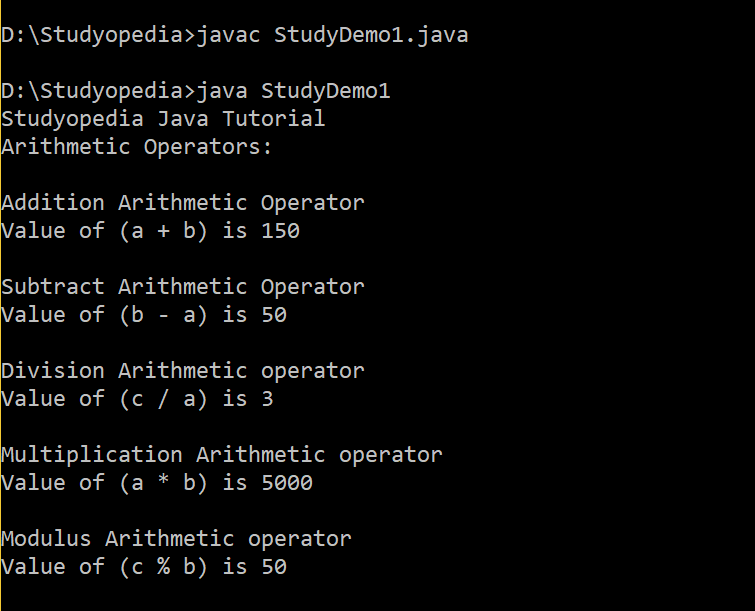## Java Assignment Operator

Use the Assignment Operator when you need to assign a value to a variable. Assignment means assigning the value of right operand(s) to the left operand.

Assignment OperatorNameExample
=Assignmenta = b

## Java Arithmetic Assignment Operator

Use the Arithmetic Assignment Operator when you need to perform arithmetic operations such as add, subtract, divide, multiple, etc and at the same time assign a value.

Arithmetic Assignment OperatorNameExample
+=Add AND assignment a += b is same as a = a + b
-=Subtract AND assignmenta -= b is same as a = a – b
*=Multiply AND assignmenta *= b is same as a = a * b
/=Divide AND assignmenta /= b is same as a = a / b
%=Modulus AND assignmenta %= b is same as a = a % b

Here’s an example,

Here’s the output,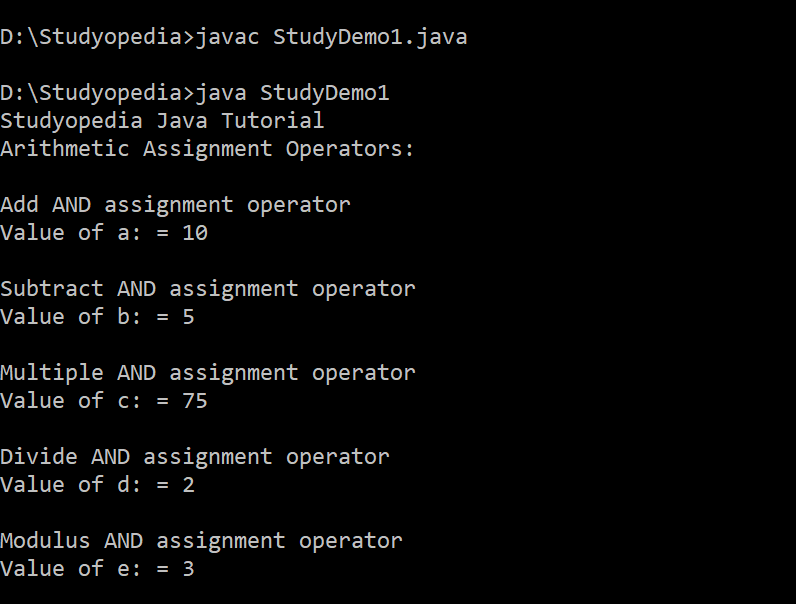## Java Relational/ Comparison operators

Compare two values with Java relational operators, which is also known as Comparison Operators.

Java Relational OperatorNameExample
= ='Equala == b isn’t true
!=Not Equala != b returns true
Greater Thana > b isn’t true
Less Thana < b is true
>=Greater than or equal toa >= b isn’t true
<=Less than or equal toa <= b is true

Here’s an example,

Here’s the output,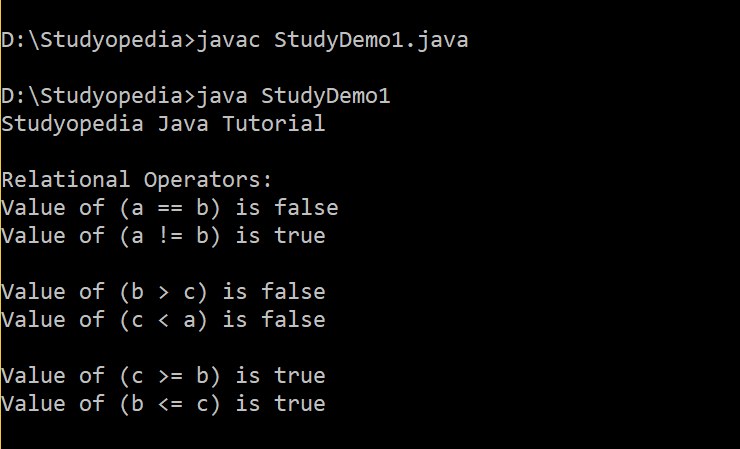## Java Logical operators

Logical operators combine conditional statements. Considering Boolean variables a and b,

Java Logical OperatorsNameExample
&&Logical AND operatora && b is true if a and b are both true
||Logical OR Operatora || b is true if either a or b is true
^Logical XOR operatora xor b is true if either a or b is true (not both)
!Logical NOT Operator!a is true if a is not true

Here’s the output,

Here’s the output,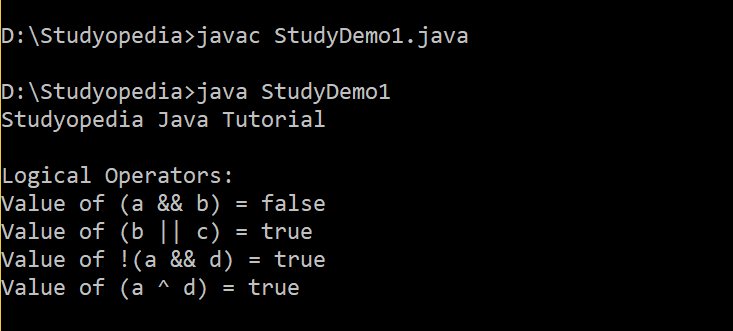## Java Unary operators

Unary operators include pre as well as post increment and decrement operators,

Here’s the example,

Here’s the output,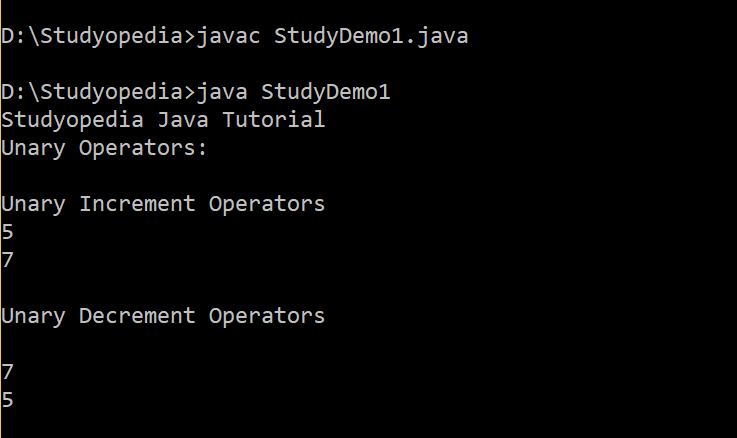## Java Ternary/ Conditional Operator

Conditional operator evaluates Boolean expressions, with has three operands. It is also known as the ternary operator. The role is to assign a value to a variable, from two given set options.

Here’s how to use it,

Here’s an example,

Here’s the output,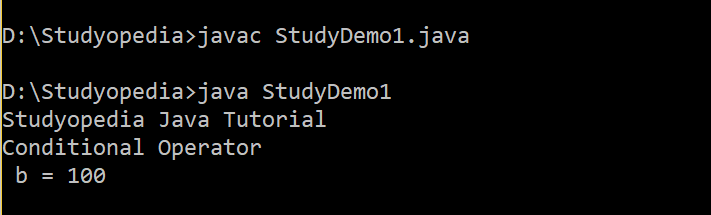## Bitwise Operator

These operators only work with the following data types,

• byte
• short
• int
• long
• char

Bitwise operators are discussed in the next lesson.

##### Tags:[email protected]

We work to create free tutorials for all.